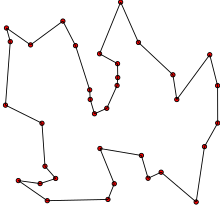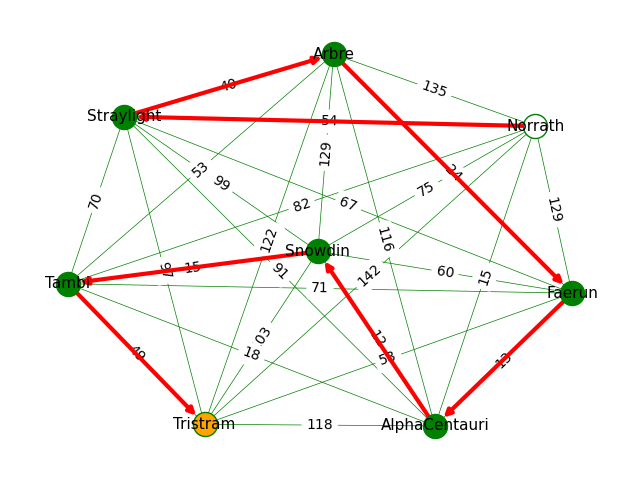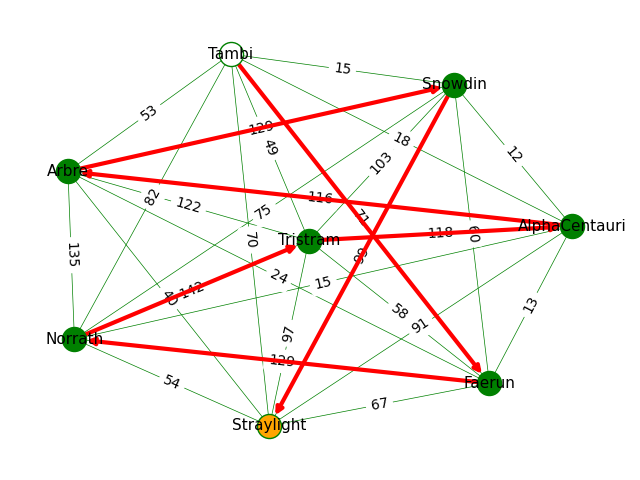# Learning Python with Advent of Code Walkthroughs

Dazbo's Advent of Code solutions, written in Python# Advent of Code 2015 - Day 9

## Problem Intro

We’re told that Santa needs to visit every location on his list exactly once. The distances between all pairs of locations have been provided, in the form of data that looks like this:

``````London to Dublin = 464
London to Belfast = 518
Dublin to Belfast = 141
``````

## Part 1

Starting at any location and ending at any location, what is the shortest distance that Santa must travel to visit every location exactly once?

This is actually a fairly standard problem, known as the Travelling Salesman Problem.

My strategy is as follows:

• Use regex to create a `(location_1, location_2):distance` dictionary entry for each distance in the input data. We’ll also store the pair of locations in reverse, so we can lookup either way.
• We then create a `set` to store all unique locations. Iterate through each location in the location pairs, and build our set of unique locations. Using a `set` makes it easy to automatically throw away locations we’ve seen before.
• Then we use `itertools.permutations()` to obtain all possible location permutations for all the locations we’ve stored in our set. For example, imagine we had just three locations, called `A`, `B`, and `C`. The `itertools.permutations()` function would return the following permutations for these three locations: `ABC, ACB, BAC, BCA, CAB, CBA`. I.e. with 3 locations, we’ll end up with `3! = 6` permutations. With 4 locations, we’ll end up with `4! = 24` permutations. And so on.
• For efficiency, we’ll filter out any permutations which are simply the reverse of an existing permutation. For example, if know the total distance for `ABC`, then we have no need to determine the distance for `CBA`, since it is the same.
• For each unique permutation, we find the distances between each pair of locations in the permutation. For example, if we have a permutation `ABC`, then we need the distance for `A -> B`, and the distance from `B -> C`.
• We add up these distances, which gives us a total journey distance for this permutation. We store this total journey distance.

After we’ve obtained the total distance for each permutation, we simply need to find the shortest distance. This is trivial, since we can just use the `min()` function and pass in our `list` of distances.

The code looks like this:

``````from pathlib import Path
import time
import re
from itertools import permutations

SCRIPT_DIR = Path(__file__).parent
INPUT_FILE = Path(SCRIPT_DIR, "input/input.txt")
# INPUT_FILE = Path(SCRIPT_DIR, "input/sample_input.txt")

def main():
with open(INPUT_FILE, mode="rt") as f:

locs_to_distances = get_distances(data) # E.g. (A, B) = n

# build our set of unique locations
locations = set()
for loc_pair in locs_to_distances:

journey_distances = [] # store total journey distances

# Create permutations of all possible combinations of locations
# I.e. all possible ways of ordering the locations we must visit.
# E.g. if we have to visit places A, B and C, there would be 3! perms:
# ABC, ACB, BAC, BCA, CAB, CBA
for loc_perm in permutations(locations):
# For efficiency: filter out inverse routes. E.g. we want ABC, but not CBA; they are the same
if loc_perm < loc_perm[-1]:
journey_dist = 0
for i in range(len(loc_perm)-1):
# iterate through location pairs i, i+1, for all locations in this permutation
# E.g. for A, B, C, we would have pairs: A-B, and B-C.
pair_a = loc_perm[i]
pair_b = loc_perm[i+1]
dist = locs_to_distances[(pair_a, pair_b)]
journey_dist += dist

# Just store the total distance for this journey.
# If we cared about the order of places, we could use a dict and store those too
journey_distances.append(journey_dist)

print(f"Shortest journey: {min(journey_distances)}")

def get_distances(data) -> dict:
""" Read list of distances between place_a and place_b.
Return dict that maps (A,B)->dist x, and (B,A)->dist x.

Args:
data (list[str]): distances, in the form "London to Dublin = 464"

Returns:
dict: (start, end) = distance
"""
distances = {}
distance_match = re.compile(r"^(\w+) to (\w+) = (\d+)")

for line in data:
start, end, dist = distance_match.findall(line)
dist = int(dist)

# create a distance record in the form: [(loc_1, loc_2), dist]
# And also store it in reverse, so that when we look it up,
# it doesn't matter which order the locations come in the journey.
distances[(start, end)] = dist
distances[(end, start)] = dist

return distances

if __name__ == "__main__":
t1 = time.perf_counter()
main()
t2 = time.perf_counter()
print(f"Execution time: {t2 - t1:0.4f} seconds")
``````

Simples!

## Part 2

I love it when we get a Part 2 like this!

We’re told that Santa wants to show off and take the route with the longest distance. Our code only needs one extra line of code! We just add this:

``````    print(f"Longest journey: {max(journey_distances)}")
``````

The final output is:

``````Shortest journey: 207
Longest journey: 804
Execution time: 0.0460 seconds
``````

That’s pretty swift!

## Solving with NetworkX

NetworkX is a cool library that allows us to build a graph, and then solve problems with that graph, e.g. shortest and longest path between two points. It can also be used to visualise our graph visually.

Here’s a solution using NetworkX…

``````from itertools import permutations
from pathlib import Path
import time
import re
import networkx as nx
import matplotlib.pyplot as plt

SCRIPT_DIR = Path(__file__).parent
INPUT_FILE = Path(SCRIPT_DIR, "input/input.txt")
# INPUT_FILE = Path(SCRIPT_DIR, "input/sample_input.txt")

DISTANCE = "distance"

SHOW_GRAPH = True

def main():
with open(INPUT_FILE, mode="rt") as f:

graph = build_graph(data)

locations = graph.nodes
journey_distances = {}

for route in permutations(locations): # E.g. for route ABC
# Use path_weight to get the total of all the edges that make up the route
route_distance = nx.path_weight(graph, route, weight=DISTANCE)
journey_distances[route] = route_distance

# Get (journey, distance) tuples
min_journey = min(journey_distances.items(), key=lambda x: x)
max_journey = max(journey_distances.items(), key=lambda x: x)

print(f"Shortest journey: {min_journey}")
print(f"Longest journey: {max_journey}")

if SHOW_GRAPH:
draw_graph(graph, min_journey)
draw_graph(graph, max_journey)

def draw_graph(graph, route):
start_node = route
end_node = route[-1]

pos = nx.spring_layout(graph) # create a layout for our graph

# Draw all nodes in the graph
nx.draw_networkx_nodes(graph, pos,
nodelist=route[1:-1], # exclude start and end
node_color="green")

# Draw all the node labels
nx.draw_networkx_labels(graph, pos, font_size=11)

# Draw start and end nodes
nx.draw_networkx_nodes(graph, pos, nodelist=[start_node],
node_color="white", edgecolors="green")
nx.draw_networkx_nodes(graph, pos, nodelist=[end_node],
node_color="orange", edgecolors="green")

# Draw closest edges for each node only - with thin lines
nx.draw_networkx_edges(graph, pos,
edge_color="green", width=0.5)

# Draw all the edge labels - i.e. the distances
nx.draw_networkx_edge_labels(graph, pos, nx.get_edge_attributes(graph, DISTANCE))

# Draw the edges that make up this particular route
route_edges = list(nx.utils.pairwise(route))
nx.draw_networkx_edges(graph, pos, edgelist=route_edges,
edge_color="red", width=3, arrows=True)

ax = plt.gca()
plt.axis("off")
plt.tight_layout()
plt.show()

def build_graph(data) -> nx.Graph:
""" Read list of distances between place_a and place_b.
Return dict that maps (A,B)->dist x, and (B,A)->dist x.

Args:
data (list[str]): distances, in the form "London to Dublin = 464"

Returns:
dict: (start, end) = distance
"""
graph = nx.Graph()
distance_match = re.compile(r"^(\w+) to (\w+) = (\d+)")

# Add each edge, in the form of a location pair
for line in data:
start, end, distance = distance_match.findall(line)
distance = int(distance)

return graph

if __name__ == "__main__":
t1 = time.perf_counter()
main()
t2 = time.perf_counter()
print(f"Execution time: {t2 - t1:0.4f} seconds")
``````

• If it were not for the function that creates a visual image of our routes, this code would be quite a bit shorter than my first solution. This is because the NetworkX package contains a number of utility methods which save us from doing many things manually.
• We’ve replaced the `get_distances()` function with the `build_graph()` function.
• It still uses regex to retrieve all the edges, i.e. as a pair of locations and the distance between them.
• But here we create a complete NetworkX `Graph` object by simply adding each new edge to the graph directly. We then return the graph object.
• As before, we then need to iterate through all permutations of the locations. This is easy to do, since we can retrieve all the locations (nodes) by simply using the `graph.nodes` attribute. Recall that each permutation of locations is a `route`.
• For each `route` we then use `nx.path_weight()` to determine the overall distance for all the edges in this route. This saves us having to get all the edges, and from having to then get the distance for each edge. Quite a few lines of code saved here!
• I’ve then stored the resulting distance in a `dict`, where the key is the `route` itself.
• Finally - for Part 1 - we use `min()` to get the shortest distance of all our routes in the dictionary. Note how I’ve used a lambda function to tell `min()` to use the values of the dict as a `key`. Our call to `min()` returns the both the route, and the route’s distance.
• Part 2 is solved in exactly the same way, except using `max()` instead of `min()`.

The output looks like this:

``````Shortest journey: (('Norrath', 'Straylight', 'Arbre', 'Faerun', 'AlphaCentauri', 'Snowdin', 'Tambi', 'Tristram'), 207)
Longest journey: (('Tambi', 'Faerun', 'Norrath', 'Tristram', 'AlphaCentauri', 'Arbre', 'Snowdin', 'Straylight'), 804)
Execution time: 0.4862 seconds
``````

This clearly runs a lot slower than my first solution. But it saved us some code, and makes it really easy to draw the graph…

### Drawing the Graph

One cool thing about NetworkX is that it’s really easy to render a visual representation of our graph. In the code above you’ll see that I’ve explicitly done the following things:

• Drawn all the nodes, except start and end.
• Drawn the start and end nodes, in a different colour.
• Drawn all the edges between pairs and label them.
• Drawn all the edges that make up the specified route, with arrows and different colour.

The resulting graphs look like this:Cool, right?